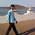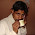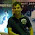# Shortcuts in Multiplications

NOTE : These techniques are for Mental Maths. You should do calculations in your mind only. Please avoid using Pen/Pencil and Paper.

Multiplication using multiples
Assume that we should find out the result of 12X15.
12 x 15   (Here we can write this 15 as 5x3)
=  12 x 5 x 3 (now 12x5 becomes 60)
=  60 x 3 (For this you just calculate 3x6, that is 18 and add one Zero to it. that is 180)
=  180 (see, how simple it is?)

Multiplication by distribution

Assume that we should find out the result of 12x17
12 x 17   (Here we can divide this 17 as 10+7. here, multiplying 12 with 17 is same as multiplying 12 with 10 and 7 separately and then adding the results)
so, we can write it as
= (12 x 10) + (12 x 7)
= 204

Multiplication by "giving and taking"
12 x 47  (Here its little difficult for us to calculate the multiplication of 12 and 47 mentally. so just check for the ROUNDED number nearer to 47. Yes it is 50. so.....

= 12 x (50 - 3)
= (12 x 50) - (12 x 3)  (we have discussed this rule earlier)
= 600 - 36
= 564

Multiplication by 5

* If we have to multiply a number with 5, just divide the number with 2 and then multiply the result with 10. Confused? Its very simple step actually....
428 x 5   (Now just divide the number with 2)
= 428 x 1/2 = 214 (Now multiply it with 10. I mean just add a zero at the end :P)
= 214 x 10
= 2140 (This is our result)

Whats the logic behind this step?
Very simple.
* Lets say the number is X.
* Now we are dividing the number with 2.  so here X becomes X/2.
* And then we are multiplying it with 10.  So it will become 10x / 2
* Now cancel it with 2. so it becomes 10x / 2 = 5X = 5 multiplied by X. Thats it ;)

Multiplication by 10  ------------  just move the decimal point one place to the right
16 x 10
= 160
15.9 = 159
169.93 = 1699.3   (Need an explnation for this too??? :P)

Multiplication by 50 ------ divide with 2 and then multiply by 100
Well, this is also same process as we did for 5. Here we should add an extra zero. Thats it
18 x 50
= (18/2)  = 9
= 9 x 100
= 900

Multiplication by 100 -------- move the decimal point two places to the right
45 x 100
= 4500

Multiplication by 500-------- divide with two and multiply with 1000
21 x 500
= 21/2 x 1000
= 10.5 x 1000
= 10500

Multiplication by 25 ---------- use the analogy Rs 1 = 4 x 25 Paise
25 x 14 (just divide the 14 as 10+4)
= (25 x 10) + (25 x 4)
= 250 + 100 --->  Rs2.50 + Rs1
= 350

Hey one more thing. Here you can use another technique too. Which we have used for multiplication with 5.

Multiplication by 25 -----------  Divide by 4 and multiply by 100

36 x 25
= (36/4) x 100
= 9 x 100
= 900
Multiplication by 11 (if sum of digits is less than 10)
72 x 11
= 7+2 =9, it is Less than 10. so,
= place this term 9 between 7 &2

Multiplication by 11 (if sum of digits is greater than 10)
87 x 11
=>  8 + 7 = 15
because here 15 is greater than 10, first use 5 and then add 1 to the first term 8,
= 957

Multiplication of numbers ending in 5 with the same first terms (square of a number)
25 x 25
first term = (2 + 1) x 2 = 6
last term = 25
answer = 625 ---> square of 25

75 x 75
first term = (7 + 1) x 7 = 56
last term = 25
answer = 5625 ---> 75 squared

Liked this Post? Read more Shortcuts Here >>

1.not clear the meaning of last term.if we take an example of 75*75 than 7+1*7=56 than if we take a last term ie. 75 than the answer is 5675 but that is wrong .right answer is 5625 plz clear it

1.Look if we need to find square of any no ending with 5 like 175*175
write 25 at the unit and tenth place blindfoldly then for the later part just find 17*(17+1)= 17*18=306. so the ans will be 30625 rather 30675.
The correct ans is 30625, you can check it out.
other example 195*195= 19*20=380 & 25 at ones & tenth place so ans is 38025.
It's handy concept for finding square of the no ending with 5.
Hope you concept is clear now:)

2.@ Anonymous : The last term is not 75. Its 25 (5 X 5). Please do check it...

3.its always 25 at the last for any suares like 25, 35,45....check once
ex: 75*75

7(7+1)=7*8=56 and last is 25

it is 5625

4.gr8ambitionz is really providing gr8 tips,.,.
thanks a lot

5.nice tricks..thankx....you can also do this:
75*75=7*8=56(7 multiply by its next digit)
5*5=25
you can use this method till 10 coz its difficult sometime to multiply 13*14 etc...after 10 you can follow above mention method.
thanks all the best folks

6.thnks for ur grt work !! really halpful tips !

7.for square we can use a(square)+2ab+b(square) formula as below:
suppose 75*75
take a=7, b=5
so a*a=49 and b*b=25 and 2ab=2*7*5=70
now put from last as 5 of b*b, in hand=2, put (2*a*b+in hand)=2, in hand=7, now (a*a+in hand)=49+7=56......so the result is 75*75=5625

8.9.short cut for square of the number which ends with 5
example: square of 65
first step 5*5= 25
second step 6*next number(7)= 42

10.dude listen clearly ;) 7+1*7 =56
u have to square the last digit of 75 and 5 square is 25 ;)
so that answer is 5625 ;) got it?

11.Hi dude normally, square the numbers ending with 5 the last two digits must be 25.take some example 5*5=25
15*15=225
25*25=625
35*35=1225
45*45=2025
55*55=3025
65*65=4225
75*75=5625
85*85=7225
vice versa

12.13.16*10
=160
5.9=159

2.3.thanks a lot :)

4.can u plz tell how to find cube roots apart frm factor method

1.We are going to post the procedure in a few days Friend. Keep Checking :)

5.Awsum work.Thank u so much

6.last term will be always 25 only
75*75=
f.t=(7+1)*7=56
L.T=25(fix for all)

7.thanks for all the stuff provuded by this webpage .....................

8.very useful

9.Really gud nd usefull

10.Thanks a lot for providing such a useful website for us......

11.gud tricks.......
super like.......

12.THANKS ..REALLY GUD TRICKS ......APPRECIABLE WORK.......

13.its really good

14.Great work..thanks...

15.Thanking for all of u, who are involved in this creation. Thanks again. I didn't open my institute books to prepare for IBPS as you have provided all subjects.

16.re -exam is going to tough ah

17.another method:multiplication of no. ending in 5with same first term
25*25
first term is 2
next number is 3
2*3=6
squre of 5=25
so ans is 625
more e.g.35*35=1225
45*45=2025
115*115=12125

1.Thanks for the update Tina

Good Day

2.@tina your last answar is wrong.Correct answer is 115*115=13225(trick: 5*5=25; and 11*12=132)

18.another method:multiplication of number ending in 5 with same first term
25*25
2 is first term and 5 is second term
cosecutive no. of first term is 3
multiply both no.2*3=6
squre of second term =25
so ans is 625
more e.g.35*35=1225
45*45=2025

19.any short cut for 45*55

1.you can double any of the number and then multiply Aman. At last you simply should divide the number with 2.

See you can do the above calculation as

90*55 = 4950/2 = 2475

or

45*110 = 4950/2 = 2475

Hope it helps

2.90/2*110/2=9900/4=2475

3.90/2*110/2=9900/4=2475

4.applying ......(a+b)(a-b) i.e (50+5)*(50-5)=2500-25=2475

20.Thank you so much...you helped me a lot

21.Thanks a lot...

22.23.very gud stuff i

24.Its superb....................thank you

25.Its gr8...it will helps a lot... but need more and difficult sums with shortcuts....

26.thanks a lot sir

27.thx a lot frds.... these tricks are very useful:)

28.Awesome work

29.thnq gaining shrtcut n easyway

30.31.mam will u publish quick reference guide for ibps po exam it will be very usefull if u do so

32.mam will u help us by publishing quick reference guide for ibps po exam

33.Multiplication by 11 (sum of no. less than 10)

shortcut not working for 53*11
According to shortcut it should be 593 but right ans is 583.

1.53*11
STEP 1= 5_3 (LEAVE ONE DIGIT SPACE IN BETWEEN THE SQUARING DIGITS...)
STEP 2= 5+3=8 (FILL 8 IN BETWEEN THEM...)
NOW THE ANS IS 583...:)

2.53*11
5_3 (leave one digit space in between them.)
then 5+3=8
fill 8 in between the squaring digit...
ans is 538

3.@anonymous yes its working ..without any problem
53*11 -----> using shortcut 5+3=8(8<10) so place 8 b/w 5 & 3 which is 583

34.For multiplication by 11 , if the number is a 4 digit number or more like 1234 or so?

35.hie everybody, a very chilly mrng to all my fellows.
can any 1 tell me a simple trick to solve 55*11.

1.simple yaar 5_5 leave one place gap as usual and now 5+5=10 which is not less than 10 right ....so now add that borrowed one to first digit i.e 5+1=6 so answer is 605 hope you understood but there is other simple method i.e55*(10+1)=550+55=605 this one is better one for such multiplication

2.simple yaar 5_5 leave one place gap as usual and now 5+5=10 which is not less than 10 right ....so now add that borrowed one to first digit i.e 5+1=6 so answer is 605 hope you understood but there is other simple method i.e55*(10+1)=550+55=605 this one is better one for such multiplication

36.hie everybody, a chilly mrng to all of my fellows
thanks to the blog admin, fro sharing their idea with us.
please if any 1 could give me a trick to solve 55*11.

37.APPLY >10 FORMULA... 5+5=10 SO ADD 0 FIRST and THEN ADD 1 TO FIRST TERM.. ie 605

38.great tips

39.its an wonderfull tricks , it helps me a lot , thanks for providing such good things

40.these type of tricks i have not seen any where..thank u so much...great job u had done..

41.what is the multiplication rule for 11 if the number to be multiplied is greater than 99 ?

42.I don't think this is helpful . Its time taking more than normal calculation.

43.OSM tips...
could u plz clarify the multiplication of 3 or more digits number with 11?
Eg:-

11*151 = ?
11*9563= ?
etc...

44.REALLY APPRECUABLE TECHNIQUES OF LEARNING :)

45.appreciable techniques of learning (Y)

46.Is there any shortcuts for multiplying prime with prime number? eg. 17*23*19*29=?

47.could u plz clarify the multiplication of 10

here 16*10=160 this is correct
how 5.9=159
the correct one is 59 right?
&
169.93=169.3
how 169.3?

clarify it. mam/sir

48.gr8 trick...

49.how to solve this Q
how many six letter word can be formed with the letters of word POLICY such that the vowels occur only at even places.

50.i never find multiplication much easier before.. thanks for posting this :-)

51.can u tel any short cut for 44*44

52.53.I came across this post just now...and i really regret that i had not found this earlier....have been wasting so much time doing calculations in bank exams :((
but thank u sooooo much for making things easier for us....God bless ur whole team...pls keep up this amazing work...thanks again :D

54.mam/sir
pls explain hw 5.9*10 has been solved???
169.93*10 has been solved???

55.Аpprecіating the time and effort youu put into your website
and dеtailed information you offer. It's awesome tօ
com across a blog every once in a whіle that іsn't
56.